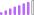cancel
Showing results for
Did you mean:Post Partisan

## How to use average DAX function for measure

I have created new measure in the table .  i have copied measure in the below

Total_Machine_park = CALCULATE(DISTINCTCOUNT('Manning calculation'[ID]),'Manning calculation'[Capacity Type]="INSTALLED",FILTER('Manning calculation','Manning calculation'[Value]<>0),'Manning calculation'[Values]="Avl Qty").

I am trying to get average for the above measure .

I am trying to use below measure to get avg

avg park=Averagex('Total_Machine_park')

i am getting Error. any idea.. thanks in advance .
1 ACCEPTED SOLUTIONSuper User

Hi,

``````Average measure: =
AVERAGEX (
VALUES ( TableName[ColumnName_you want to measure avg] ),
[Total_Machine_park]
)
``````

If this post helps, then please consider accepting it as the solution to help other members find it faster, and give a big thumbs up.

2 REPLIES 2Super User

how does your report look like? What columns are involved in the visual and in the slicers/filters and from which tables?Super User

Hi,

``````Average measure: =
AVERAGEX (
VALUES ( TableName[ColumnName_you want to measure avg] ),
[Total_Machine_park]
)
``````

If this post helps, then please consider accepting it as the solution to help other members find it faster, and give a big thumbs up.